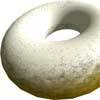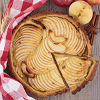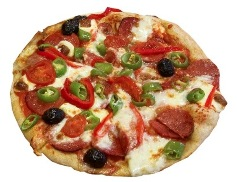#### You may also like### Doughnut

How can you cut a doughnut into 8 equal pieces with only three cuts of a knife?### Pies

Grandma found her pie balanced on the scale with two weights and a quarter of a pie. So how heavy was each pie?### Red Balloons, Blue Balloons

Katie and Will have some balloons. Will's balloon burst at exactly the same size as Katie's at the beginning of a puff. How many puffs had Will done before his balloon burst?

# Pizza Portions

## Pizza Portions

My friends and I love pizza.This is great because we always know what kind of food to make. There is a small problem. We often end up arguing over how much each person has eaten. We like to be fair and share out the pizzas equally.

Can you help us?

If I had $1$ pizza and wanted to share it equally between $2$ of us, how much pizza would we get each?

How much would each of us have if we had $1$ pizza to share equally between 3 people?

Sometimes, we have some pizza left over which we reheat the next day.

If I have $1/2$ a pizza and I want to split it between $2$ of us, what fraction of the whole pizza do we each get?

If there were $1/3$ of a pizza left and $2$ of us to share it, what fraction of the whole pizza would we get now?
How about if there were $3$ of us and we had $1/4$ of a pizza to share?

Look back at what you've done so far. Can you write down how you worked out your answers using numbers and maths symbols?

Can you see any patterns in the numbers that you've used?

Today, each of us would like $1/2$ a pizza. If I have $1$ pizza, how many people can have $1/2$ a pizza?

If I had $2$ pizzas altogether how many people can have $1/2$ a pizza each now?

Can you think of a way to write this using numbers and symbols?

Can you see any patterns in the numbers this time?

Sometimes, we may be a little hungrier so we'd like $2/3$ of a pizza each.

How many pizzas would I need to buy for $3$ people?

How many for $4$ of us? Would there be any left over?

How could you write this using numbers and symbols?

### Why do this problem?

Doing this activity gives pupils the opportunities to gain more understanding and better recognising of simple fractions of a whole. They also can be relating fractions to division i.e. understanding that finding one third is the same as dividing by 3. Somethimes when children pursue activities like this they start being able to divide fractions themselves i.e. one quarter split into two equal pieces. It can also be a starting point for beginning to divide by a fraction i.e. How many halves in 1?
Also there is an element of recognising, explaining, generalising and predicting number patterns.

### Possible approach

It is important that children understand first how to express fractions - the idea of splitting one whole into more than one equally sized pieces. Drawings of some kind are essential at this stage. Circular pizzas could be cut out of (gummed) paper which children could then fold into different fractions. Discuss how to divide a circle into equal sized parts:
Does it matter where we fold/draw the lines?
What is important about these lines?
This pictorial representation may be useful all the way through this activity.
Each stage of this investigation can be extended to consolidate the ideas.
When asking pupils to write down mathematical expressions, you may like to go right back to using just whole numbers. For example:
How could I write $4$ pizzas shared between $2$ people?
So can you tell me how we could write $1$ pizza shared between $2$?
What about $1/2$ pizza shared between $2$?
In the third and fourth sections, children may offer division or multiplication sums and this can lead into interesting discussion in itself about the relationship between the two operations.